# pls do all Page 6 of 14 22) Identify a good buffer. ) small amounts of both a weak acid and its conjugate base B) significant amounts of both a strong acid and a strong bso C) small amounts of bot... related homework questions

• #### pls do all Page 6 of 14 22) Identify a good buffer. ) small amounts of both a weak acid and its conjugate base B) significant amounts of both a strong acid and a strong bso C) small amounts of bot...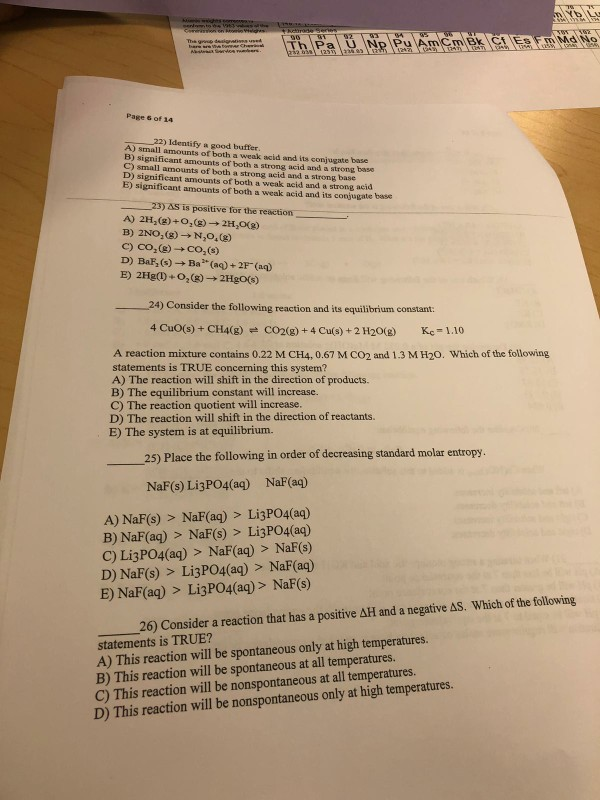pls do all Page 6 of 14 22) Identify a good buffer. ) small amounts of both a weak acid and its conjugate base B) significant amounts of both a strong acid and a strong bso C) small amounts of both a strong acid and a strong base D) significant amounts of both a weak acid and a strong acid E) significant amounts of both a weak acid and its conjugate base...

• #### This reaction is classified as: Strong Acid + Strong Base, Weak Acid + Strong Base, Strong Acid + Weak Base, Weak Aci...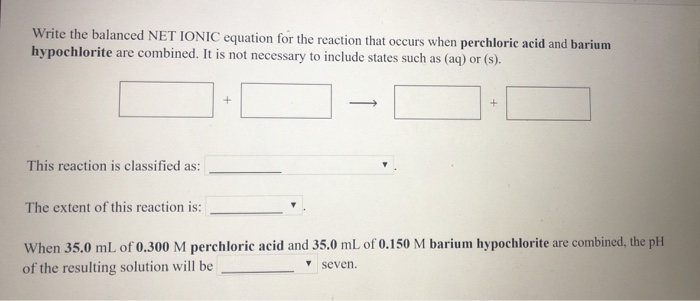This reaction is classified as: Strong Acid + Strong Base, Weak Acid + Strong Base, Strong Acid + Weak Base, Weak Acid + Weak Base. The extent of this reaction is: Below 50%, 50%, Above 50%, 100% When 35.0 mL of 0.300 M perchloric acid and 35.0 mL of 0.150 M barium hypochlorite are combined, the pH of the resulting solution will be greater than, equal to,...

• #### Question 39 1 pts A weak acid (HA) reacts with a weak base (B) to form the conjugate base of the acid (A) and the conjugate acid of the base (B-H the pKa of HA was 4.8 and the pKa of BH* (i.e., the p...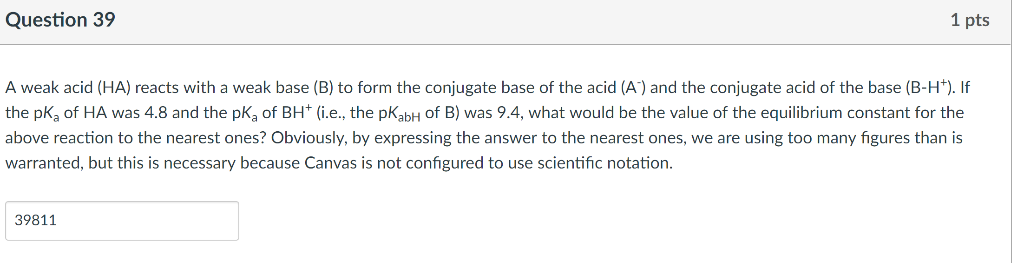Question 39 1 pts A weak acid (HA) reacts with a weak base (B) to form the conjugate base of the acid (A) and the conjugate acid of the base (B-H the pKa of HA was 4.8 and the pKa of BH* (i.e., the pKabH of B) was 9.4, what would be the value of the equilibrium constant for the above reaction to the nearest ones? Obviously, by expressing the answer to the n...

• #### 8. Identify the following as a strong acid, a strong base, a weak acid, a weak base, or a neutral...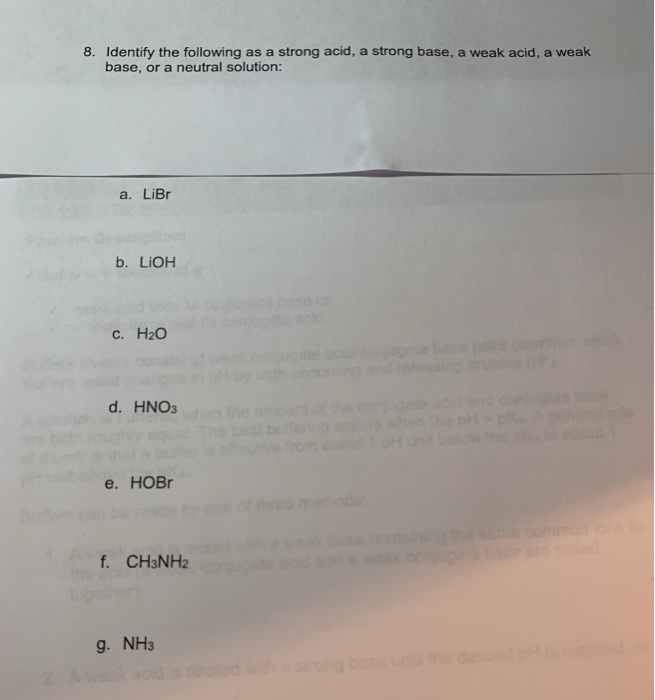8. Identify the following as a strong acid, a strong base, a weak acid, a weak base, or a neutral solution: a. LiBr b. LiOH c. H20 d. HNO3 e. HOBr f. CH3NH2 g. NH3 8. Identify the following as a strong acid, a strong base, a weak acid, a weak base, or a neutral solution: a. LiBr b. LiOH c. H20 d. HNO3 e. HOBr f. CH3NH2 g. NH3

• #### A. Match each type of titration to its pH at the equivalence point. Weak acid, strong base Strong acid, strong base...

A. Match each type of titration to its pH at the equivalence point. Weak acid, strong base Strong acid, strong base Weak base, strong acid pH less than 7 pH equal to 7 pH greater than 7 B. A 56.0 mL volume of 0.25 M HBr is titrated with 0.50 M KOH. Calculate the pH after addition of 28.0 mL of KOH. C. Consider the titration of 50.0 mL of 0.20...

• #### ± pH of a Strong Acid, a Weak Acid, a Strong Base, and a Weak Base Part A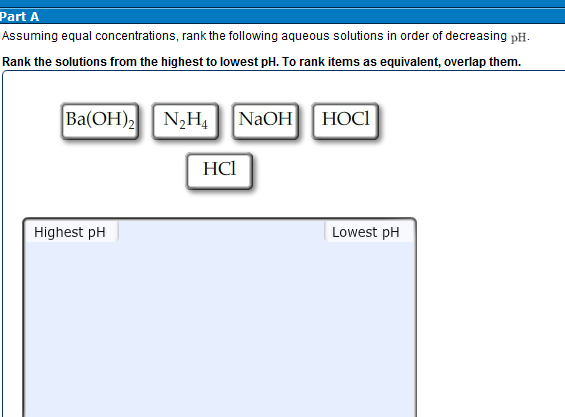• #### ± pH of a Strong Acid, a Weak Acid, a Strong Base, and a Weak Base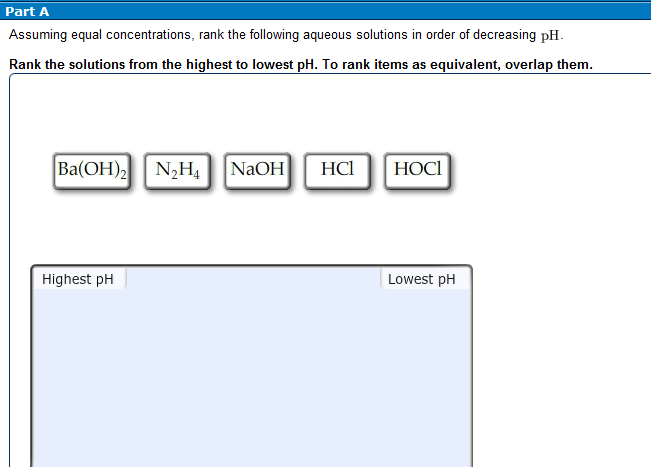• #### Part A: Classify each substance as a strong acid, strong base, weak acid, or weak base. Drag the appropriate items to t...Part A: Classify each substance as a strong acid, strong base, weak acid, or weak base. Drag the appropriate items to their respective bins. LiOH, HF, H2SO4, CH3COOH, HClO4, NaOH, Ca(OH)2, NH3, HBr, HCOOH, CsOH, HNO2, HI, HCN, KOH, (CH3)2NH, Ba(OH)2, HNO3, HCl, CH3NH2 Strong Acid Weak Acids Strong Bases Weak Bases Part B: What salt is produced in each...

• #### why is the pH at end point for a titration of a weak acid with a strong base is greater than 7, yet that of the titration of a strong acid with a strong base is equal to 7?

why is the pH at end point for a titration of a weak acid with a strong base is greater than 7, yet that of the titration of a strong acid with a strong base is equal to 7?

• #### pKa Conjugate base Acid formula Conjugate base name Acid name F3CCOOH 0.20 F3ccoo trifluoroacetic acid trifluoroacetate...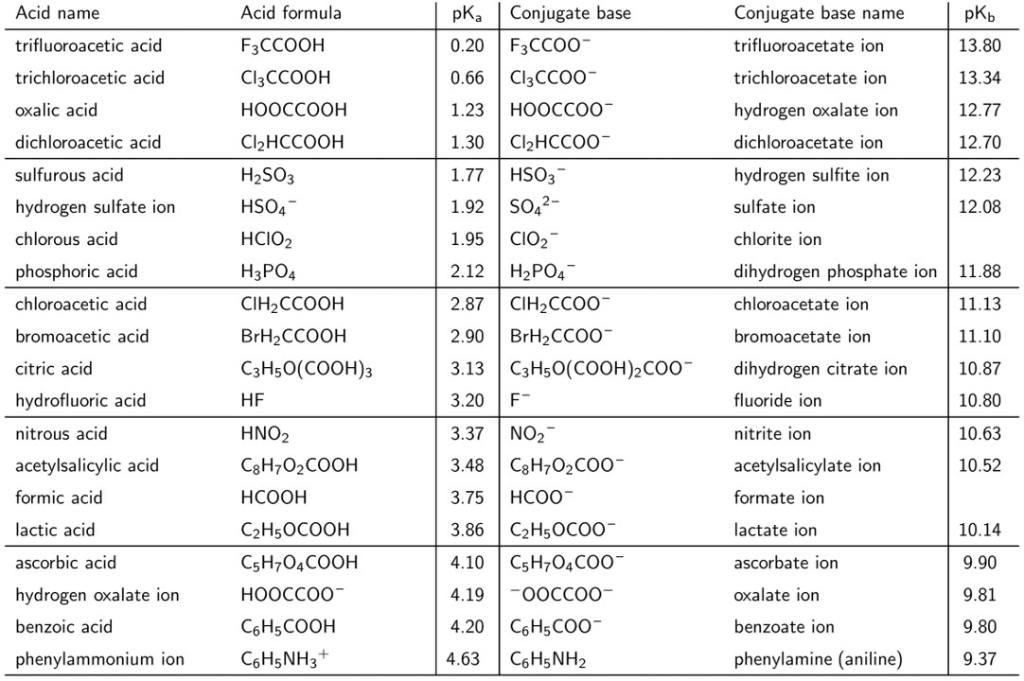pKa Conjugate base Acid formula Conjugate base name Acid name F3CCOOH 0.20 F3ccoo trifluoroacetic acid trifluoroacetate ion 13.80 Cl3CCOOH 13.34 0.66 Cl3CCOO trichloroacetic acid trichloroacetate ion HOOCCOOH 1.23 HOOCCOO oxalic acid hydrogen oxalate ion 12.77 Cl2HCCOOH 12.70 dichloroacetic acid 1.30 Cl2HCCOO dichloroacetate ion H2SO3 12.23 1.77 HSO3 sulfur...

• #### In pous solution, classify these compounds as strong acids, weak acids, strong buses, or weak hases. Strong acid Wea...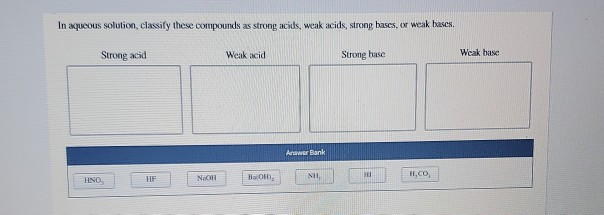In pous solution, classify these compounds as strong acids, weak acids, strong buses, or weak hases. Strong acid Weak acid Strong hase Weak base Arower Bank HISTO, HF NaOH BOND E .CO

• #### name acid, base, conjugate acid, and conjugate base

Name each of the following species for the following acid-base reactions. (The equilibrium lies to the right in each case, i.e., the product side is favored. If thespecies is an ion, include the word "ion" in the name. Use systematic names such as "methanol" instead of archaic names like "methyl alcohol" or "wood alcohol".)a. CH3CH2O- (ethoxide ion) + H3O+ (...

• #### #58 Olle reactants 15 e U w (b) the conjugate base of the acid, and (c) the conjugate éj acid of the base. HCO3 + HC...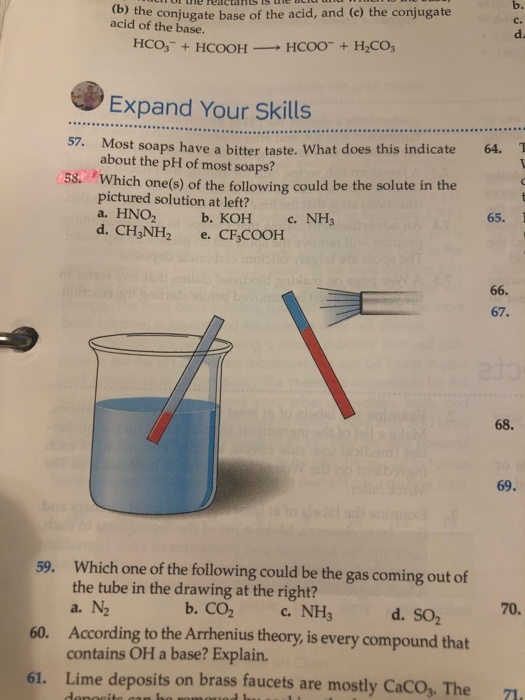#58 Olle reactants 15 e U w (b) the conjugate base of the acid, and (c) the conjugate éj acid of the base. HCO3 + HCOOH — HCOO + H2CO3 Expand Your Skills 64. 58 Most soaps have a bitter taste. What does this indicate about the pH of most soaps? Which Which one(s) of the following could be the solute in the pictured solution at left? a. HNO2 b. KOH C. NH3...

• #### If a buffer solution is 0.520 M in a weak acid (Ka-5.4 x10 and 0.220 M in its conjugate base, wha...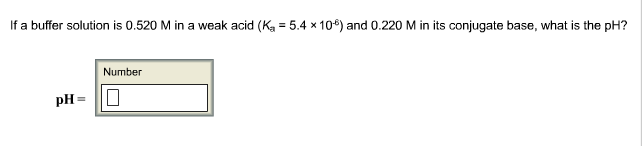If a buffer solution is 0.520 M in a weak acid (Ka-5.4 x10 and 0.220 M in its conjugate base, what is the pH? Number If a buffer solution is 0.520 M in a weak acid (Ka-5.4 x10 and 0.220 M in its conjugate base, what is the pH? Number

• #### If a buffer solution is 0.120 M0.120 M in a weak acid (?a=7.5×10−5)Ka=7.5×10−5) and 0.440 M0.440 M in its conjugate base...

If a buffer solution is 0.120 M0.120 M in a weak acid (?a=7.5×10−5)Ka=7.5×10−5) and 0.440 M0.440 M in its conjugate base, what is the pH?

• #### 1 . If a buffer solution is 0.260 M in a weak acid (?a=8.3×10−5)and 0.480 M in its conjugate base, what is the pH? pH= 2...

1 . If a buffer solution is 0.260 M in a weak acid (?a=8.3×10−5)and 0.480 M in its conjugate base, what is the pH? pH= 2. If a buffer solution is 0.200 M in a weak base (?b=5.0×10−5) and 0.530 M in its conjugate acid, what is the ph 3. Phosphoric acid is a triprotic acid (?a1=6.9×10−3, ?a2=6.2×10−8 , and ?a3=4.8×10−13 To find the pH of a buffer composed of H...

• #### If a buffer solution is 0.300 M in a weak base (K5 = 2.7 x 10 ) and 0.400 M in its conjugate acid, what is the pH?...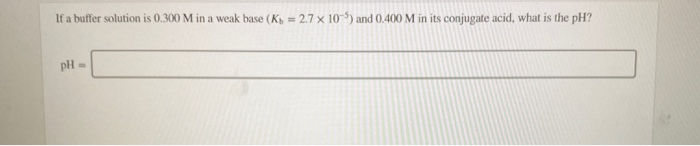If a buffer solution is 0.300 M in a weak base (K5 = 2.7 x 10 ) and 0.400 M in its conjugate acid, what is the pH? pH = You need to prepare 100.0 mL of a pH 4.00 buffer solution using 0.100 M benzoic acid (pK-4.20) and 0.200 M sodium benzoate How many milliliters of each solution should be mixed to prepare this buffer? benzoic acid: Amt ml. sodium benz...

• #### For the Google page search network shown below, find the page-to-page matrix of the network. T4 T2 r6 T1 35 For the Google page search network shown below, find the page-to-page matrix of the ne...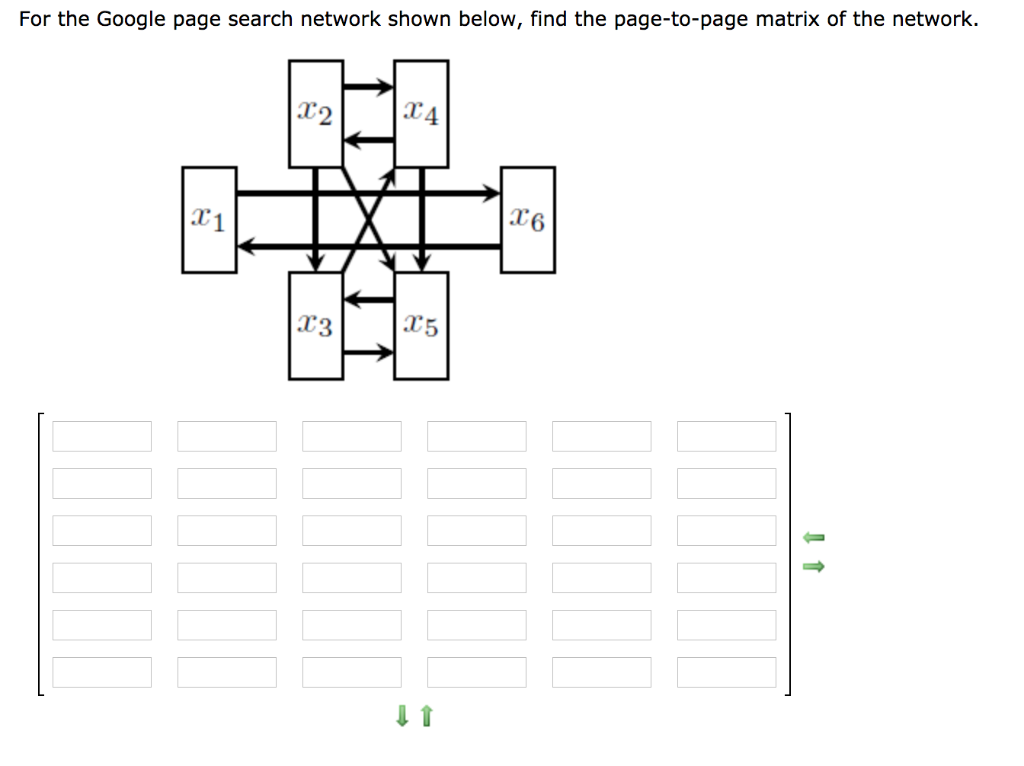For the Google page search network shown below, find the page-to-page matrix of the network. T4 T2 r6 T1 35 For the Google page search network shown below, find the page-to-page matrix of the network. T4 T2 r6 T1 35

• #### For the Google page search network shown below, find the page-to-page matrix of the network. T2 T1 T3 For the Google page search network shown below, find the page-to-page matrix of the network....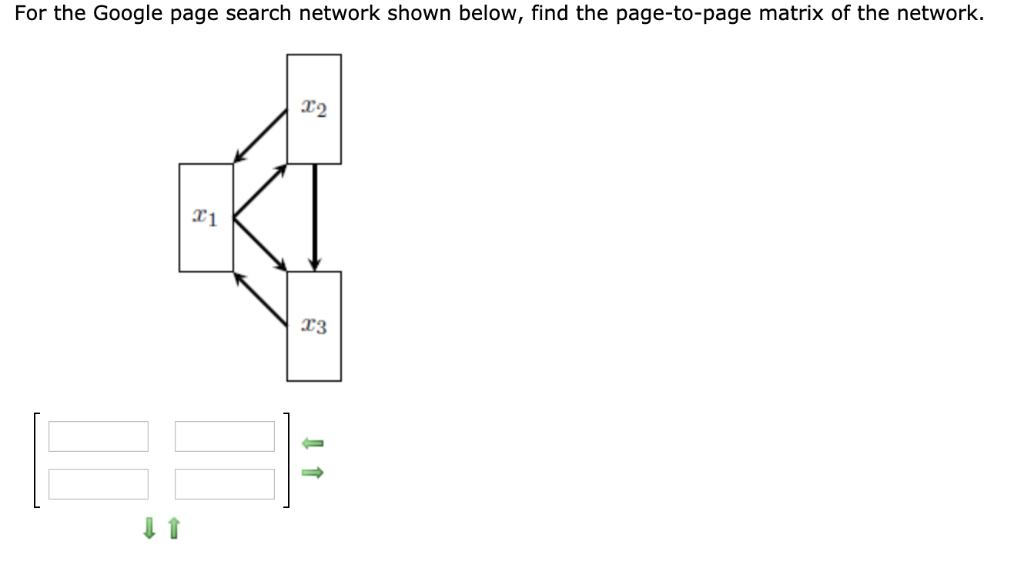For the Google page search network shown below, find the page-to-page matrix of the network. T2 T1 T3 For the Google page search network shown below, find the page-to-page matrix of the network. T2 T1 T3

• #### 25> HCIO is a weak acid (K M in NaCIO at 25 °C? 4.0 × 10-8) and so the salt NaClO acts as a weak base, what is the pH of a solution that is 004 pH = 25> HCIO is a weak acid (K M in NaCI...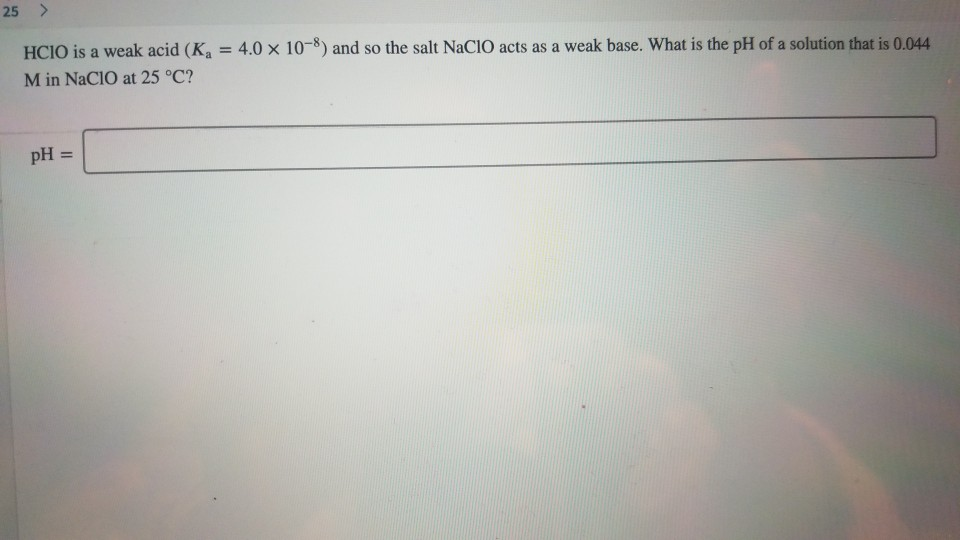25> HCIO is a weak acid (K M in NaCIO at 25 °C? 4.0 × 10-8) and so the salt NaClO acts as a weak base, what is the pH of a solution that is 004 pH = 25> HCIO is a weak acid (K M in NaCIO at 25 °C? 4.0 × 10-8) and so the salt NaClO acts as a weak base, what is the pH of a solution that is 004 pH =

Need Online Homework Help?